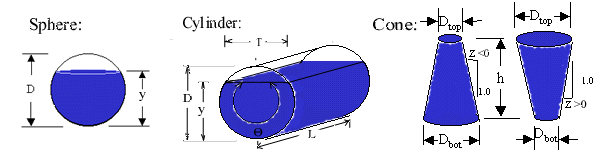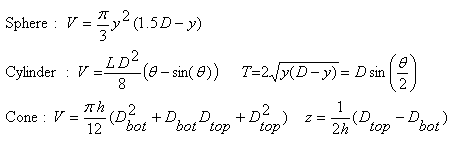Volume of partially full cylindrical, spherical, and conical tanks

Calculations and equations for partially full storage tanks:  Partially full cylindrical tank on its side, partially full spherical container, and conical shape.

Register to enable "Calculate" button.

 © 2014 LMNO Engineering, Select shape: Research, and Software, Ltd. Sphere Cylinder. Enter   y, D, L Cylinder. Enter D,T,L (<= half full) Cylinder. Enter D,T,L (> half full) Cone. Enter h, Dtop, Dbot Cone. Enter h, Dtop, z Cone. Enter h, Dbot, z http://www.LMNOeng.com Liquid Depth, y: cm m inch ft Diameter, D: cm m inch ft Liquid Top Width, T: cm m inch ft Cylinder Length, L: cm m km inch ft mile Volume, V: cm3 liter m3 km3 inch3 quart gallon ft3 bushel (U.S.) barrel yard3 Acre-ft mile3

Units in Cylinder, Cone, and Sphere Volume Calculation: barrel=42 US gallons, cm=centimeter, ft=feet, gallon=US gallon, km=kilometer, m=meterEquations for Sphere, Cylinder, and Cone Volume (Rade and Westergren, 1990)Discussion of Volume Calculation
This web page is designed to compute volumes of storage tanks for engineers and scientists; however, it may be useful to anyone who needs to know the volume of a full or partially full sphere, cylinder, or cone.  Technically, our "cone" is not really a cone; it is a "frustum of a right cone" (Rade and Westergren, 1990, p. 73).  A cone is a frustum that has a top diameter of 0.0.  For the cylinder laying on its side, if you enter liquid top width, diameter, and length, there are two possible solutions for depth and volume; therefore, when using the drop-down menu to select which calculation to perform, you must select whether the cylinder is more or less than half full.

Variables used in Volume Equations
D = Sphere or cylinder diameter [L].
Dbot = Cone bottom diameter [L].
Dtop = Cone top diameter [L].
h = Cone height [L].
L = Cylinder length [L].
T = Top width of liquid in cylinder [L].
V = Volume of liquid in cylinder, cone, or sphere [L3].
y = Liquid depth in sphere or cylinder [L].
z = Horizontal to vertical side slope of cone.  z<0 if Dtop<Dbot.  z=0 if Dtop=Dbot.  z>0 if Dtop>Dbot.
θ = Angle representing how full the cylinder is [radians or degrees].  An empty cylinder has θ=0o,  a cylinder with θ=180o is half full, and a cylinder with θ=360o is completely full.

Error Messages
"D must be > 0."  Cylinder diameter was entered as <= 0; it must be positive.
"D's must be >= 0."  Dtop or Dbot for the cone was entered as negative.
"h must be >= 0."  Cone height was entered as negative.
"L must be > 0."  Cylinder length was entered as <= 0.
"T must be >= 0."  Cylinder top width was entered as negative.
"T must be <= D."  Cylinder liquid top width cannot be greater than cylinder diameter.
"y must be >= 0."  Sphere or cylinder liquid depth was entered as negative.
"y must be <= D."  Sphere or cylinder liquid depth cannot be greater than diameter.
"Infeasible. Dbot will be < 0."  The slope that was input for the cone gives a bottom diameter that is negative, which is physically impossible.  Most likely the slope was entered with an incorrect sign.  Slope is Horizontal/Vertical and is negative for a cone with a smaller top than bottom.
"Infeasible. Dtop will be < 0."  Similar to above.

References
Rade, Lennart and Bertil Westergren. 1990. Beta Mathematics Handbook. CRC Press.  2ed.

LMNO Engineering, Research, and Software, Ltd.
7860 Angel Ridge Rd.   Athens, Ohio 45701  USA   Phone: (740) 592-1890
LMNO@LMNOeng.com    http://www.LMNOeng.com

To: